Thursday, January 23, 2020
Home > Latest Announcement > NMTC 2016 Question Papers With Solutions Primary Level For Class 5 and 6

# NMTC 2016 Question Papers With Solutions Primary Level For Class 5 and 6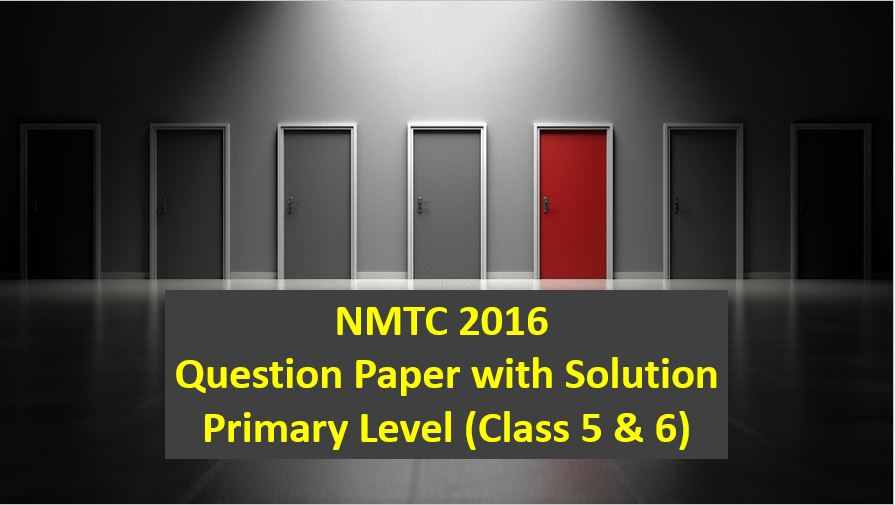# NMTC 2016 Question Papers with Solutions Primary Level

NMTC is an acronym for National Mathematics Talent Contest. NMTC is conducted by AMTI (Association of Mathematics Teachers of India) to identify the talent of the students in Mathematics. In this post, NMTC 2016 Question Papers with Solutions Primary Level is published.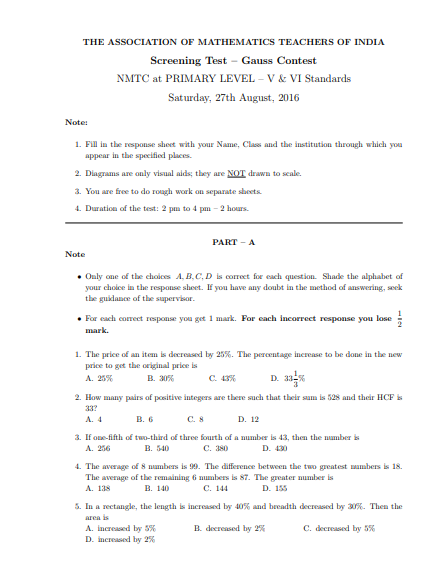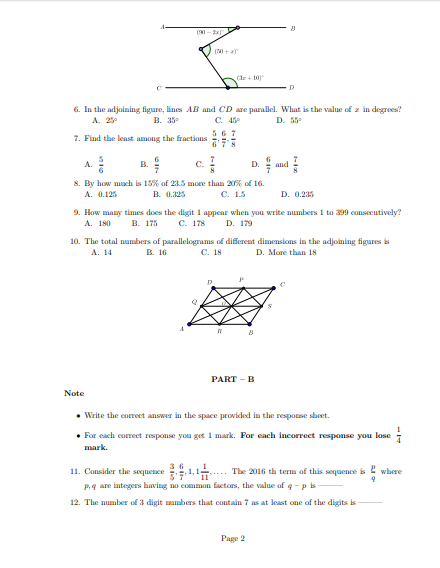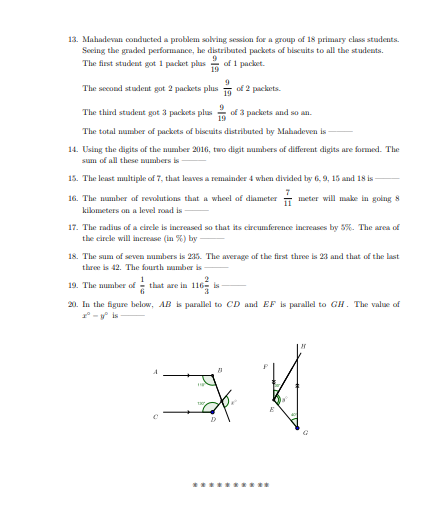Part A: Instruction:

Only One of the choices A, B, C, D is correct. For each correct response, you get 1 mark. For each incorrect response, you lose 1/2 mark.

NMTC 2016 Paper For Primary Level Ques No 1:

The price of an item is decreased by 25%. The percentage increase to be done in the new price to get the original price is

Options:

A. 25%

B. 30%

C. 43%

D. 33 1/3%

Solution: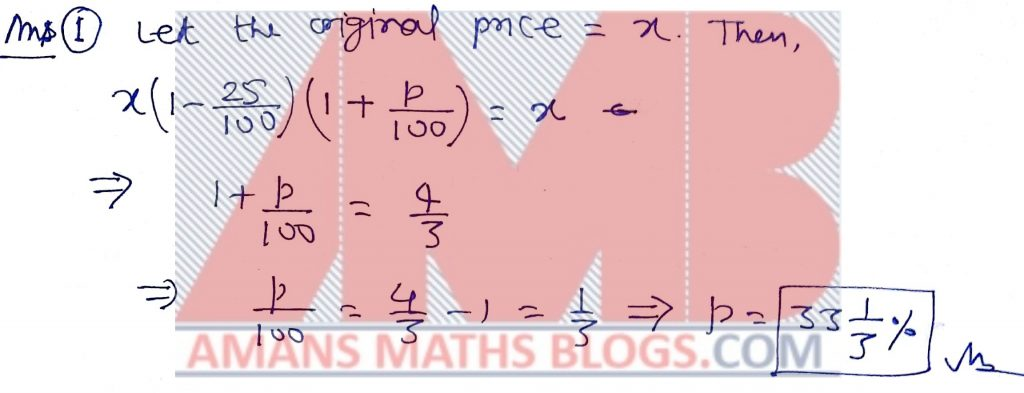NMTC 2016 Paper For Primary Level Ques No 2:

How many pairs of positive integers are there such that their sum is 528 and their HCF is 33?

Options:

A. 4

B. 6

C. 8

D. 12

Solution: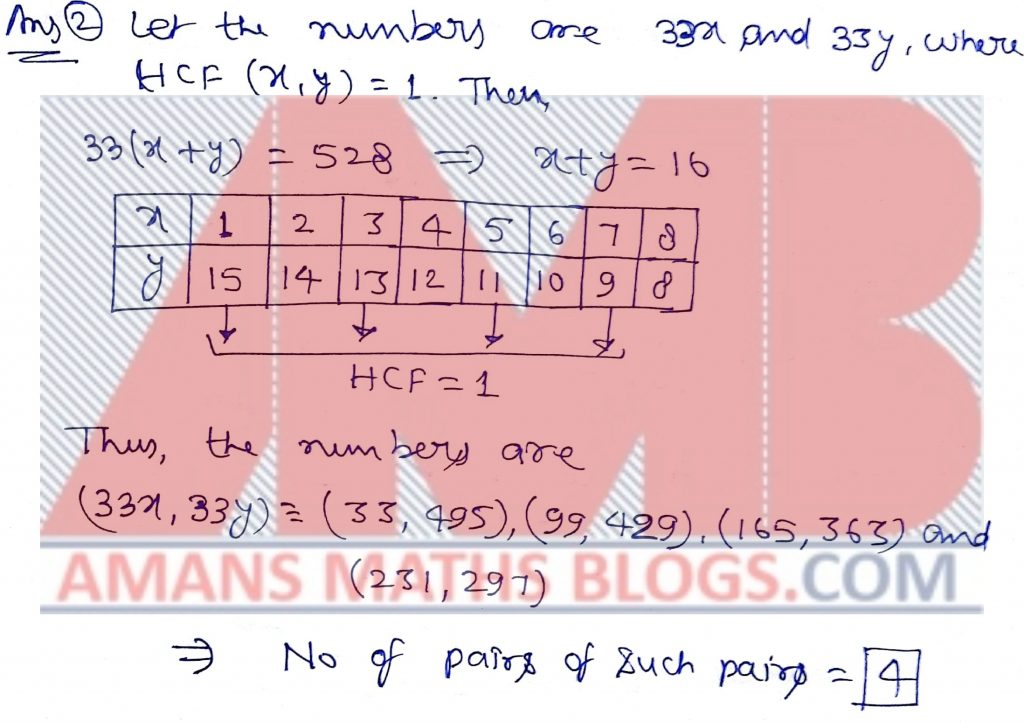NMTC 2016 Paper For Primary Level Ques No 3:

If one-fifth of two-third of three fourth of a number is 43, then the number is

Options:

A. 256

B. 540

C. 380

D. 430

Solution: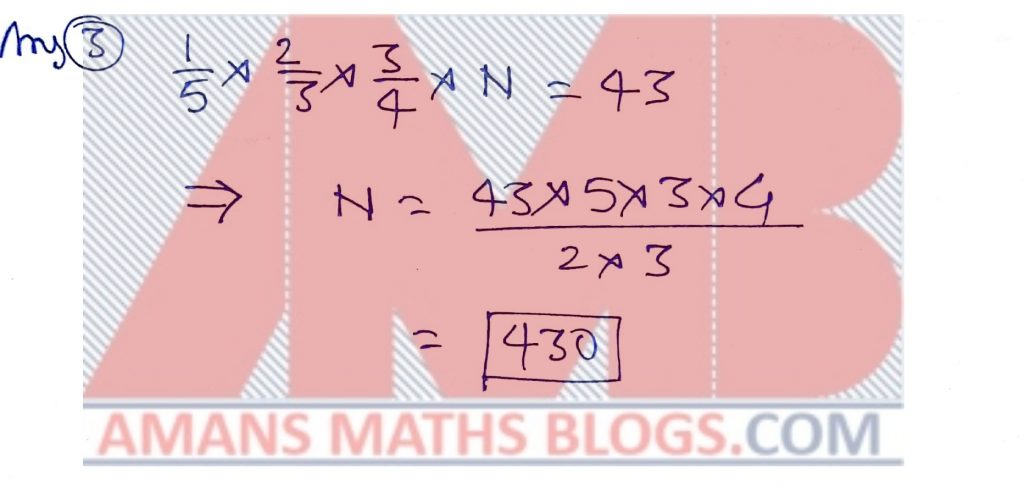NMTC 2016 Paper For Primary Level Ques No 4:

The average of 8 numbers is 99. The difference between the two greatest numbers is 18. The average of the remaining 6 numbers is 87. The greater number is

Options:

A. 138

B. 140

C. 144

D. 155

Solution:NMTC 2016 Paper For Primary Level Ques No 5:

In a rectangle, the length is increased by 40% and breadth decreased by 30%. Then the area is

Options:

A. Increased by 5%

B. Decreased by 2%

C. Decreased by 5%

D. Increased by 2%

Solution: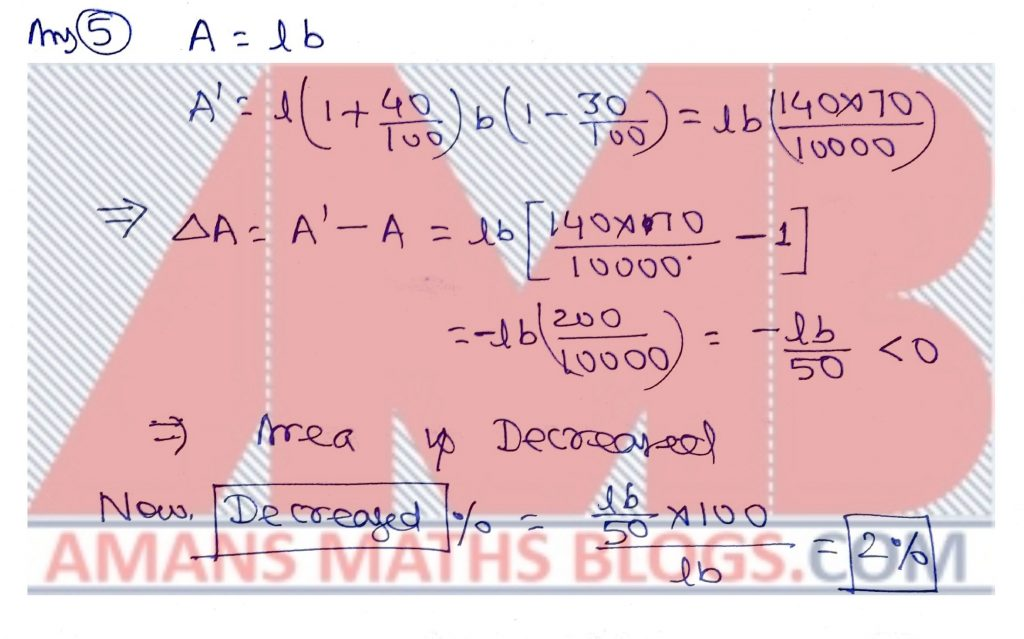NMTC 2016 Paper For Primary Level Ques No 6:

In the adjoining figure, lines AB and CD are parallel. What is the value of x in degrees?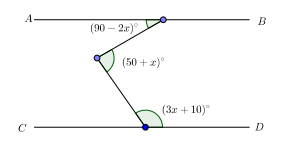Options:

A. 25 Degree

B. 35 Degree

C. 45 Degree

D. 55 Degree

Solution: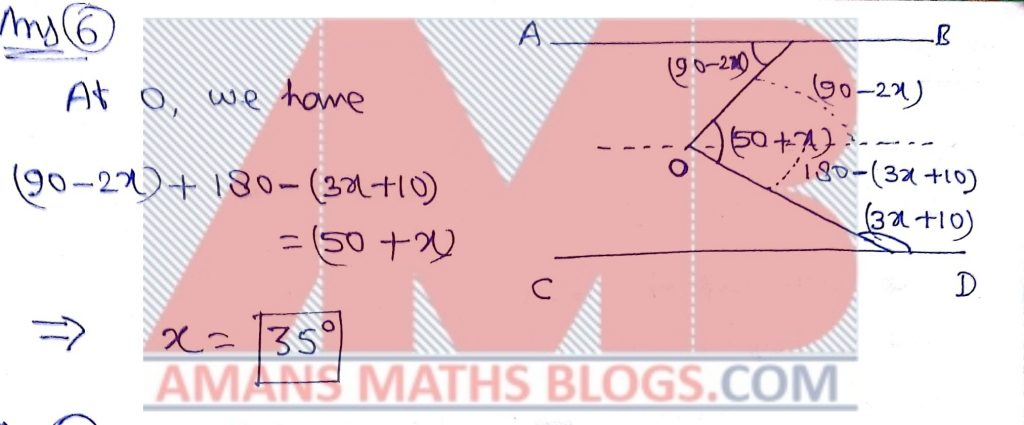NMTC 2016 Paper For Primary Level Ques No 7:

Find the least among the fractions 5/6, 6/7, 7/8.

Options:

A. 5/6

B. 6/7

C. 7/8

D. 6/7 and 7/8

Solution:NMTC 2016 Paper For Primary Level Ques No 8:

By how much is 15% of 23.5 more than 20% of 16.

Options:

A. 0.125

B. 0.325

C. 1.5

D. 0.235

Solution:NMTC 2016 Paper For Primary Level Ques No 9:

How many times does the digit 1 appear when you write numbers 1 to 399 consecutively?

Options:

A. 180

B. 175

C. 178

D. 179

Solution:NMTC 2016 Paper For Primary Level Ques No 10:

The total numbers of parallelograms of different dimensions in the adjoining figures is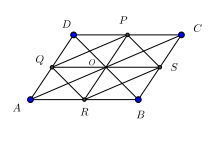Options:

A. 14

B. 16

C. 18

D. More Than 18

Solution:Part B: Instruction:

Write the correct answer in the space provided in the response sheet. For each correct response, you get 1 mark. For each incorrect response, you lose 1/4 mark.

NMTC 2016 Paper For Primary Level Ques No 11:

Consider the sequence 3/5, 6/7, 1, 1 1/11. The 2016th term of this sequence is p q where p, q are integers having no common factors, the value of q − p is _____ .

Solution: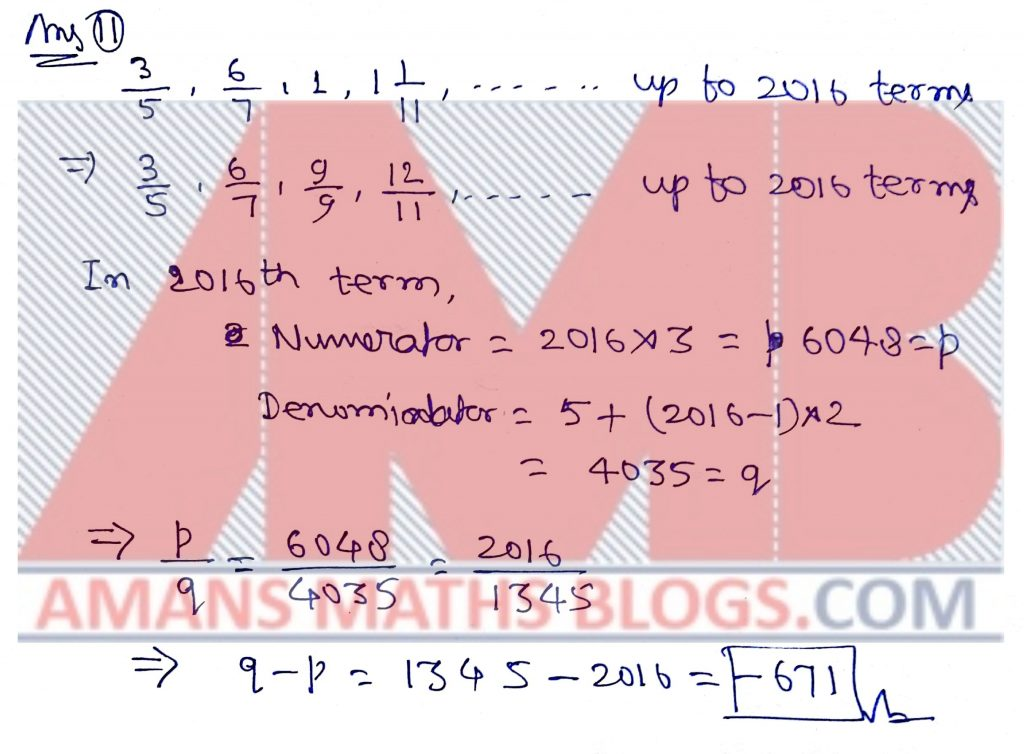NMTC 2016 Paper For Primary Level Ques No 12:

The number of 3 digit numbers that contain 7 as at least one of the digits is ______ .

Solution:NMTC 2016 Paper For Primary Level Ques No 13:

Mahadevan conducted a problem solving session for a group of 18 primary class students. Seeing the graded performance, he distributed packets of biscuits to all the students.

The first student got 1 packet plus 9/19 of 1 packet.

The second student got 2 packets plus 9/19 of 2 packets.

The third student got 3 packets plus 9/19 of 3 packets and so on.

The total number of packets of biscuits distributed by Mahadevan is ____.

Solution: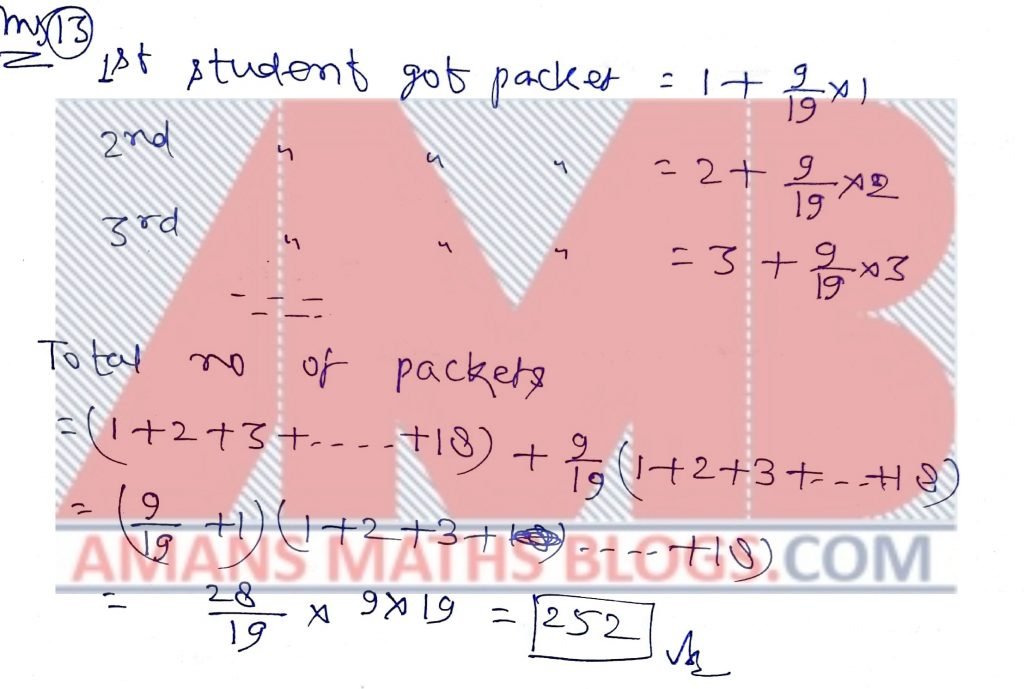NMTC 2016 Paper For Primary Level Ques No 14:

Using the digits of the number 2016, two digit numbers of different digits are formed. The sum of all these numbers is ____ .

Solution: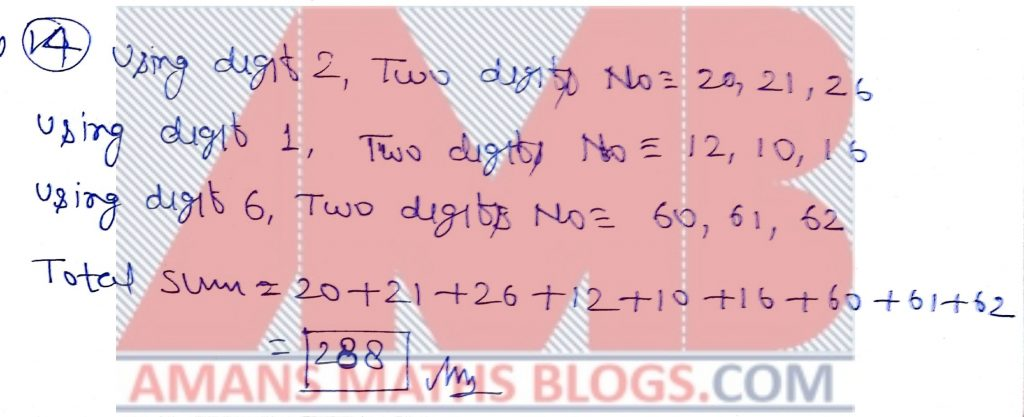NMTC 2016 Paper For Primary Level Ques No 15:

The least multiple of 7, that leaves a remainder 4 when divided by 6, 9, 15 and 18 is _____.

Solution: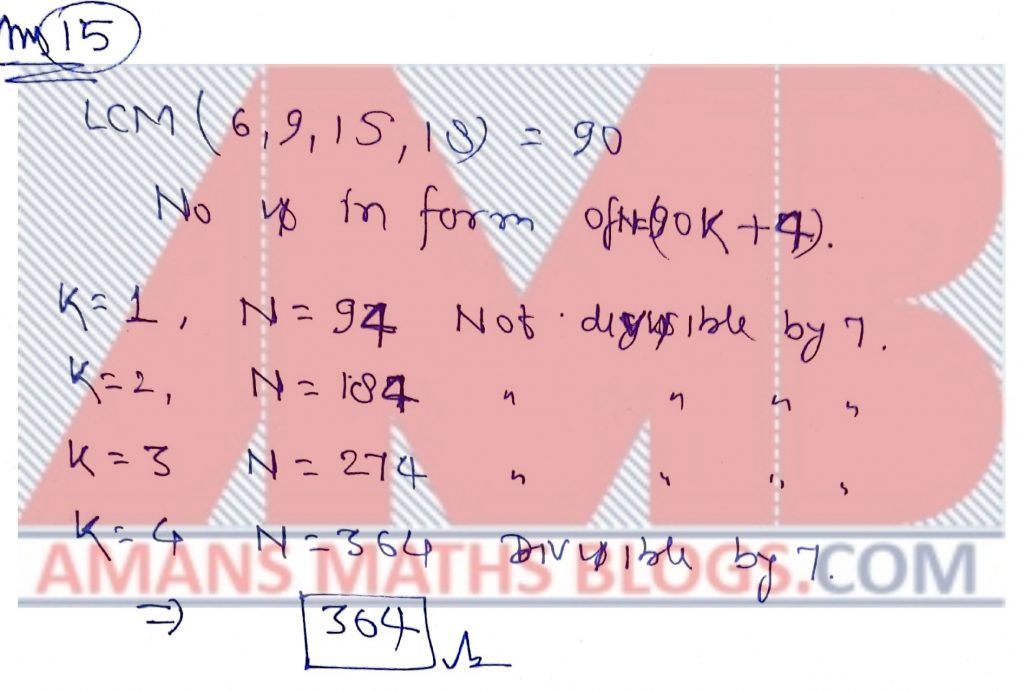NMTC 2016 Paper For Primary Level Ques No 16:

The number of revolutions that a wheel of diameter 7 11 meter will make in going 8 kilometers on a level road is

Solution:NMTC 2016 Paper For Primary Level Ques No 17:

The radius of a circle is increased so that its circumference increases by 5%. The area of the circle will increase (in %) by _____ .

Solution:NMTC 2016 Paper For Primary Level Ques No 18:

The sum of seven numbers is 235. The average of the first three is 23 and that of the last three is 42. The fourth number is _____.

Solution:NMTC 2016 Paper For Primary Level Ques No 19:

The number of 1/6 that are in 116 2/3 is ____ .

Solution: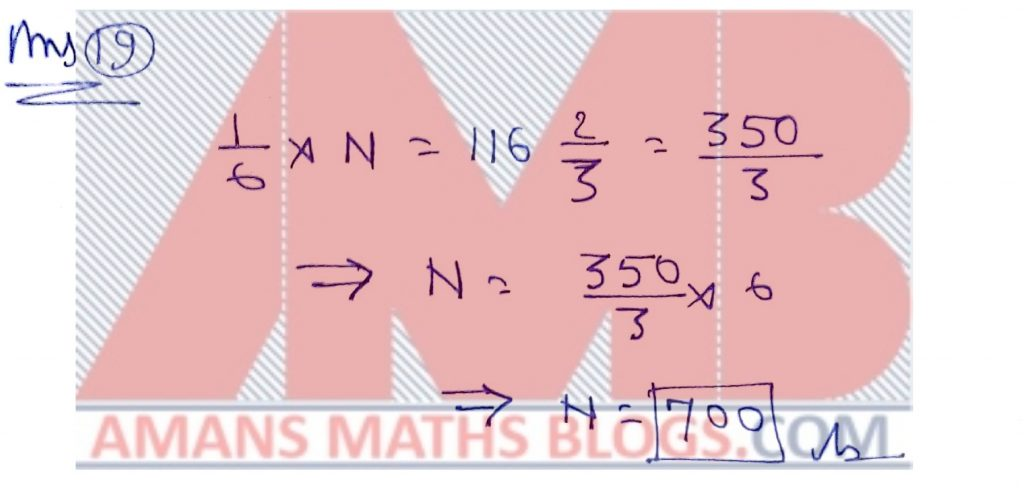NMTC 2016 Paper For Primary Level Ques No 20:

In the figure below, AB is parallel to CD and EF is parallel to GH. The value of x0 − y0 is _____.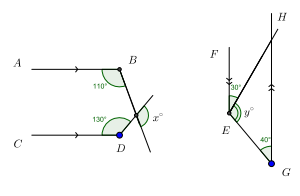Solution: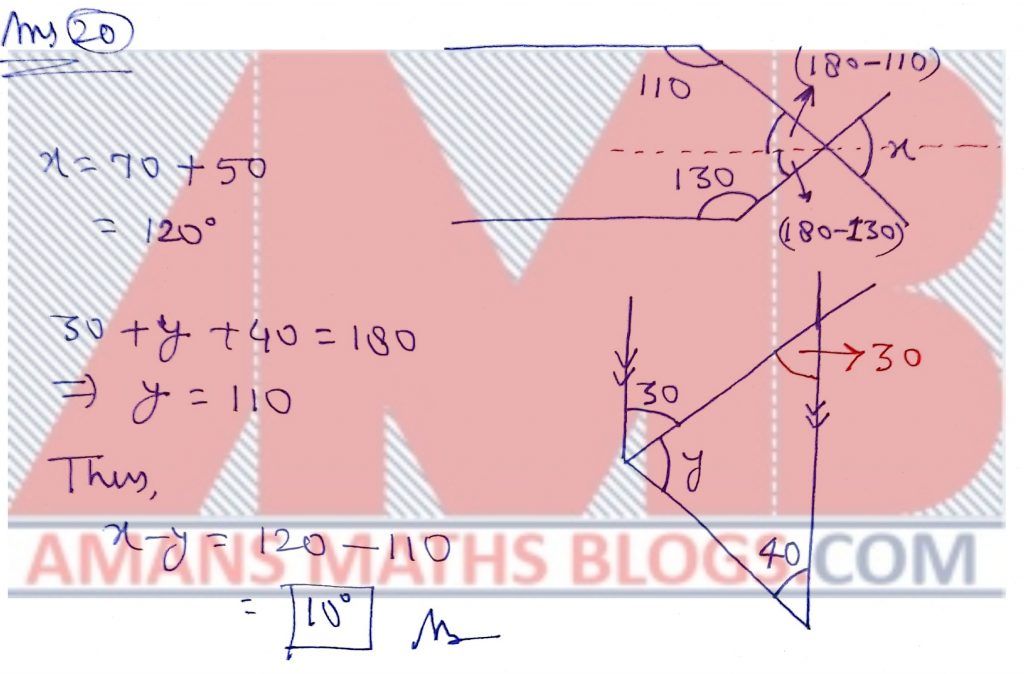1___ D

2___ C

3___ D

4___ C

5___ B

6___ A

7___ A

8___ B

9___ A

10__ C

11__ –671

12__ 252

13__ 252

14__ 255

15__ 364

16__ 4000

17__ 10.25%

18__ 40

19__ 700

20__ 10

To get NMTC 2004 to 2018 Questions with Solutions, Click Image Below.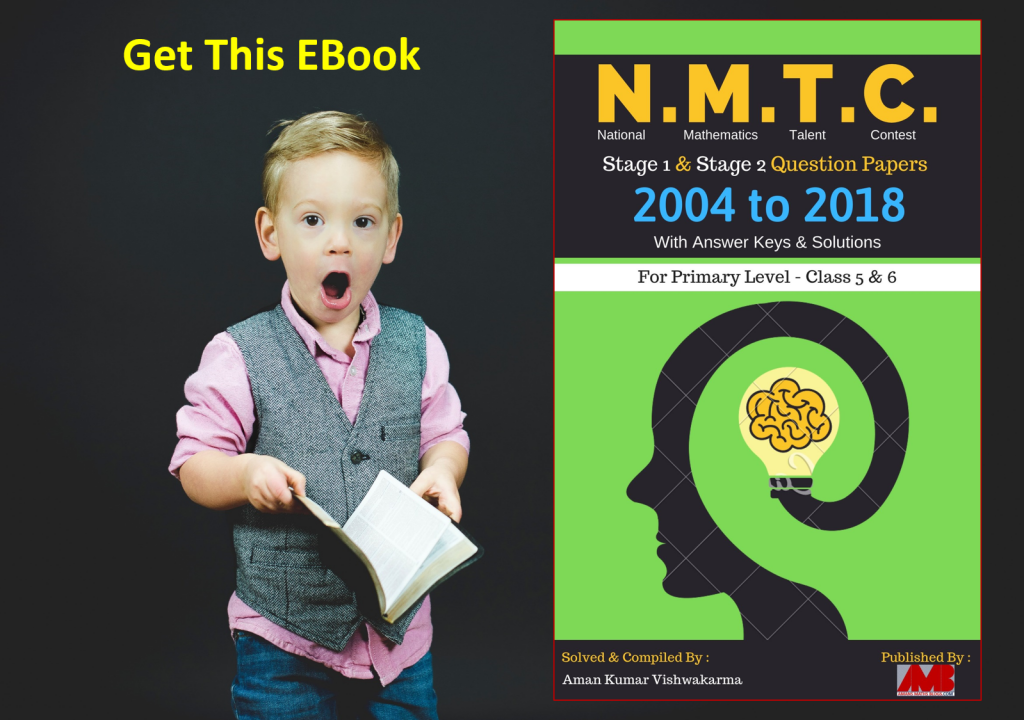Click On Image To Get EBOOK

error: Content is protected !!